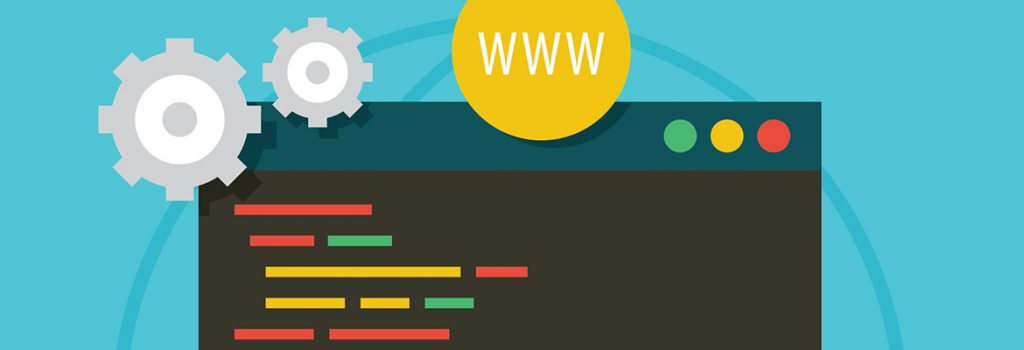## PROGRAMMING MINDSET HELP FOR BEGINNERS

February 19, 2022

### Introduction

Programming is broken down into 2 skills sets to master (logic and syntax).

Logic is the steps you take to accomplish the goals of your program. In communication, logic is what you say.

Syntax is the way that you write the program. In communication, syntax is the patterns that you use to spell words and combine them in a sentence.

Different programming languages can perform the same function (logic) but in different ways (syntax). Below is an example of different programming languages commands to print to the screen (same logic) but different syntax.

Notice that each language can have very different syntax but the logic above is the same. They will each print “Hello world!” to the screen.

When your program is not working correctly first identify if the problem is syntax, logic or both. If both then separate the syntax part of the problem from the logical part of the problem.

### Syntax Problems

A lot of beginner programmers have trouble with syntax because they are not used to seeing the syntax patterns. Knowing this will help you to focus your attention on understanding and solving these problems quickly. The nice thing about syntax errors are that the system will tell you when you have them. Here is a methodology that will help to find and fix these errors.

When you have a syntax error the system will tell you this in the form of an Error message (see example below). To solve this do the following steps:

1. Find where the syntax problem is. Sometimes that system will be incorrect where the problem is because one syntax problem can create several areas that look incorrect to the system. But you want to identify exactly what code is causing the error.
2. Once the problem is identified relook up the syntax for that function or set of code you are trying to create. I have made many a mistake because I just knew that the syntax was correct only to find out after I looked up the correct form I had been incorrect.
3. If that does not work then create a simpler version of the function or code snippet using the correct syntax so that you understand exactly how the syntax works then add more complexity to the code until you have it all worked out.

Here is a syntax error from python

Notice the red section. The system is telling you there is a syntax problem here. Compare this print statement with the one above. You should notice that the () are in front of the “Hello World” and not surrounding the text.

### Logic Problems

These can be more complicated to solve. The program is running but not giving you the correct answer or doing the right thing. A set of techniques you can use to solving logic problems are:

1. Identify that you have a problem. Understand what should be happening and then look at what is not happening. The idea is to try and narrow down what and where the logic is incorrect.
2. Make sure that the logic that you want is represented by the program that you wrote.
3. Make sure that the logic that you want is the logic that will solve the problem.
4. Break down the problem in smaller parts and making sure each of those parts work the way you think they should.

Here is a Python logic error:

Notice that this while loop will run forever.

### Summary

Overall programming is comprised of logic and syntax. Each is needed for a successful program and each has its own set of issues. Learning how to understand and solve syntax and logical issues are vital to getting a correctly working program.